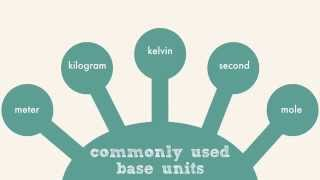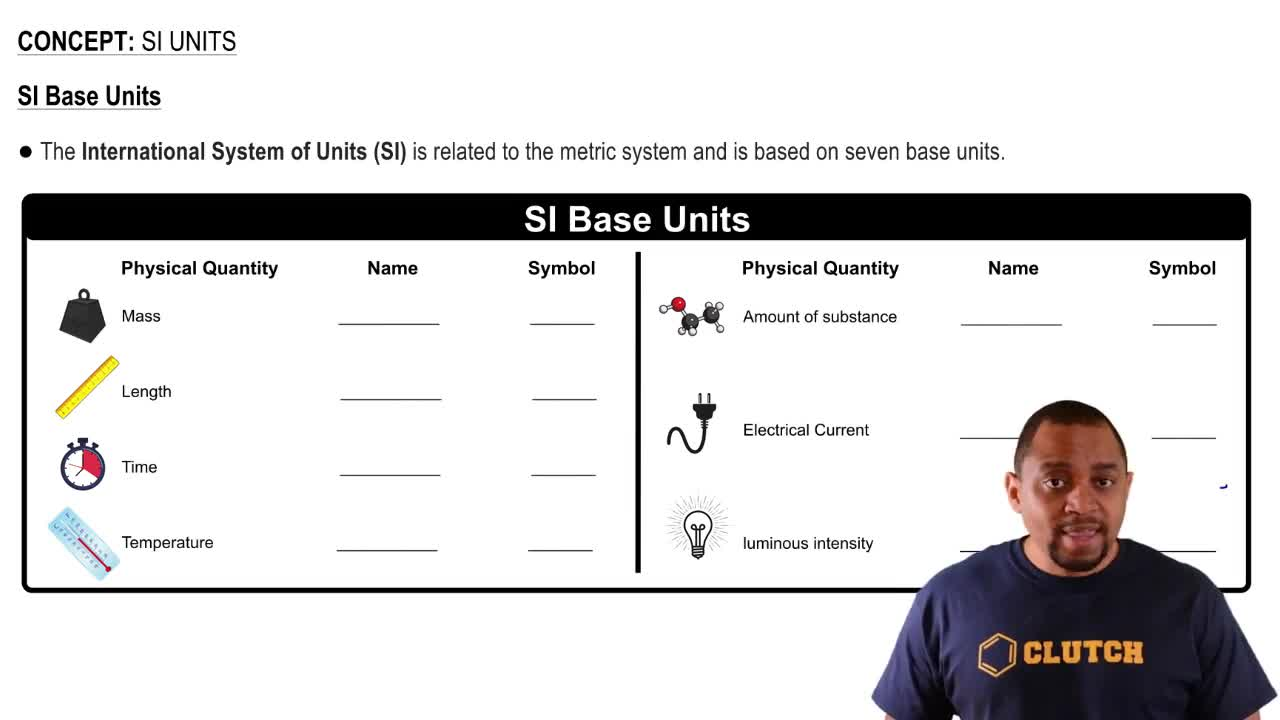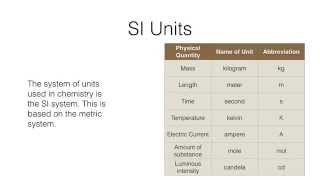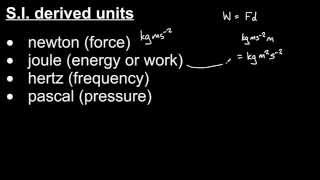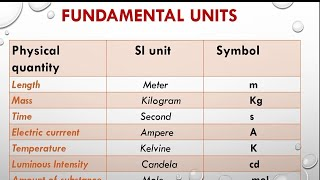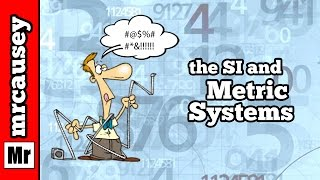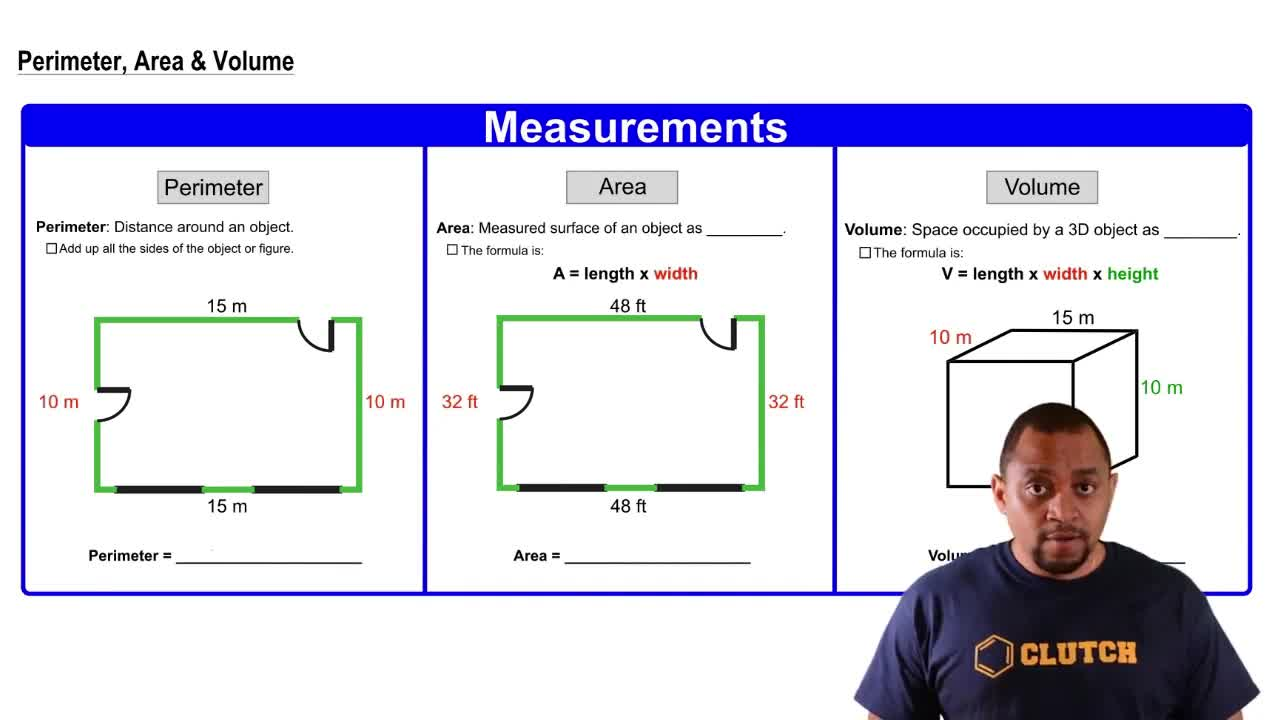Start typing, then use the up and down arrows to select an option from the list.
1. 1. Intro to General Chemistry2. SI Units
Problem

# What SI units are used for measuring the following quanti-ties? For derived units, express your answers in terms of the six fundamental units. (b) Length

Relevant Solution1m
Play a video:
Hey there. So inspect this problem, we need to state whether the following is a measurement of length, area, volume, mass density, time or temperature. Alright, so for the first one we have a number of milligrams, right? So grams is a measurement of length mass. Alright, so this is a mess. It could have been in g mg, pictograms, millie, you know, whatever it is, it's still mass 77 Calvin. This is Calvin. And Calvin is a unit of temperature. Okay? Now this is in kilometers squared. So if it was just a kilometer, then we know this is length, right? If it was a kilometer, but because this is squared, that gives us area, So this was actually area, right? So kilometer squared is an area and not length. Ah and then this is PICO s. Seconds. So obviously this is just time. Alright folks, thank you so much for watching. We'll see you in the next video.